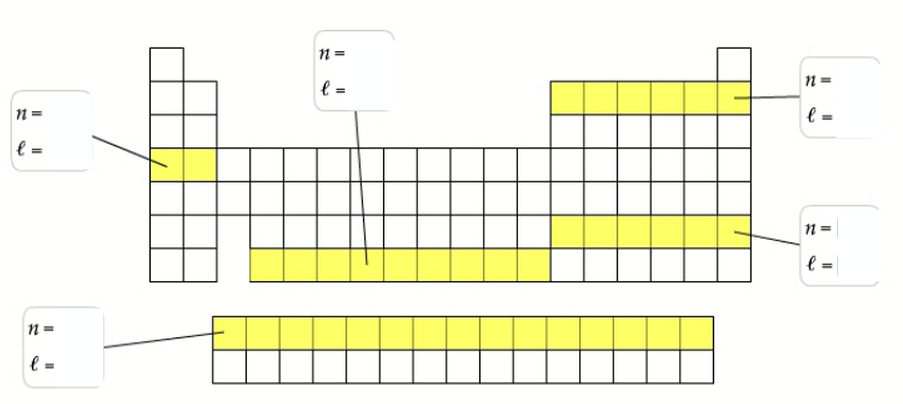# Problem: For each set of elements represented in this periodic table outline, identify the principal quantum number, nn, and the azimuthal quantum number, ℓ, for the highest energy electrons in an atom of one of those elements.The periodic table lists all known elements arranged by atomic number. Atomic number is the nuclear charge, the number of protons in the nucleus of an an atom of a particular element. For a neutral atom, the number of protons is equal to the number of electrons. Each column of the table, called a group, contains elements with the same number of valence electrons that are in different quantum levels. Each row of the table, called a period, contains elements with differing numbers of valence electrons that are in the same principal quantum level. The four main blocks of the table (s, p, d, and f) contain elements whose highest energy electrons have the same azimuthal quantum number (ℓ).

###### FREE Expert Solution
91% (498 ratings)View Complete Written Solution
###### Problem Details

For each set of elements represented in this periodic table outline, identify the principal quantum number, nn, and the azimuthal quantum number, ℓ, for the highest energy electrons in an atom of one of those elements.The periodic table lists all known elements arranged by atomic number. Atomic number is the nuclear charge, the number of protons in the nucleus of an an atom of a particular element. For a neutral atom, the number of protons is equal to the number of electrons. Each column of the table, called a group, contains elements with the same number of valence electrons that are in different quantum levels. Each row of the table, called a period, contains elements with differing numbers of valence electrons that are in the same principal quantum level.

The four main blocks of the table (s, p, d, and f) contain elements whose highest energy electrons have the same azimuthal quantum number (ℓ).